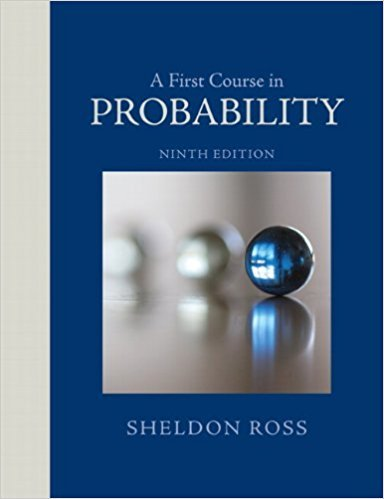×
Get Full Access to A First Course In Probability - 9 Edition - Chapter 4 - Problem 10p
Get Full Access to A First Course In Probability - 9 Edition - Chapter 4 - Problem 10p

×

# Let X be the winnings of a gambler. Let p(i) = P(X = i)ISBN: 9780321794772 63

## Solution for problem 10P Chapter 4

A First Course in Probability | 9th Edition

• Textbook Solutions
• 2901 Step-by-step solutions solved by professors and subject experts
• Get 24/7 help from StudySoup virtual teaching assistantsA First Course in Probability | 9th Edition

4 5 1 432 Reviews
12
3
Problem 10P

Problem 10P

Let X be the winnings of a gambler. Let p(i) = P(X = i) and suppose that

P(0) = 1/3; P(1) = P(-1) = 13/55;

p(2)=p(-2) = 1/11; p(3) = p(-3) = 1/165

Compute the conditional probability that the gambler

Step-by-Step Solution:

Step 1 of 1

(a)

Letbe the winnings of a gambler.

Letand suppose thatWe are asked to compute the conditional probability that the gambler winsgiven that he wins a positive amount.

Letbe the event that we win something.

We need to findUsing Bayes’ rule we can write that……(1)So that we havewhenHence the conditional probability that the gambler winsStep 2 of 1

##### ISBN: 9780321794772

Unlock Textbook Solution